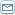# Forex Trading Using Fibonacci Retracement Zones...

 By: Sam_BeatsonWhat forex day trading signals do you use to enter and exit the market?How do you know that they are not going to give you a false entry signal?How can you use these signals to exit your trade?Let's look at Fibonacci first of all. This 750 year old "natural order" of numbers reflects the birth of rabbits in a field, the number of rinds on a pineapple, the sequence of sunflower seeds. So how do we apply it to forex trading?First of all we need to understand that Fibonacci is a commonly traded forex day trading signals indicator. The ratio given by the Fibonacci numbers are converted into a percentage. The Fibonacci sequence of numbers is 1,1,2,3,5,8,13,21,34,55,133,222 etc. adding the left number to get the next number in the sequence. When we apply Fibonacci to our charts, we take a particular market move of say 50-100 points and plot the Fibonacci ratio levels.This brings out levels of potential support and resistance on to our charts. The top of the move is considered "0%" of the move and the start of the move is considered as "100%". We then have Fibonacci "retracement" levels at 23.6%, 38.2%, 50% and 68.1%. These "retracement zones" can give us forex day trading signals.If the price has moved down say 70 pips and then retraces we can say that the strongest Fibonacci point of resistance is at 23.6% and if the price is going to stop and reverse back to the original direction after the correction. If we break the 23.6%, then the 38.2% is the next strongest resistance level then the 50%. If we hit the 23.6% resistance line and the price "bounces" back downwards, we can start thinking about whether this was just a correction - a Fibonacci retracement.It is not enough just to know the price has hit the line of resistance and bounced back though. We should also try to get an indication that the strength and momentum of the market is also in favour with our theory. For this, we could have a slow stochastic oscillator, a MACD and a RSI just as an example to give us an indication of the weight of our reentry into the trade or late entry based on the retracement idea.You would be surprised at how accurate the Fibonacci method of trading is in terms of how history repeats itself again and again in the forex market. It is very tempting to exit a trade when the price turns the other way, however it is worth utilising Fibonacci to ensure it is not a minor (23.6%) retracement and allowing the trade to run it's full course.
Most Read Articles On
"Investment"SuggestedTop Searches on

 Share this article :
Click to see more related articles